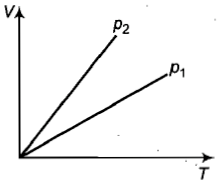You’ve reached the end of your free Videos limit.
#2-Defining-basic-terms
(Physics) > Kinetic Theory of Gases
Unable to watch the video, please try another server

#### My Notes

Create your notes while watching video by clicking on icon in video player.
Related Practice Questions :

An isolated system-

(A) is a specified region where transfers of energy and mass take place

(B) is a region of constant mass and only energy is allowed to close the boundaries

(C) is one in which mass within the system is not necessarily constant.

(D) cannot transfer either energy or mass to or from the surroundings.

High Yielding Test Series + Question Bank - NEET 2020

Difficulty Level:

Volume, pressure, and temperature of an ideal gas are V, P, and T respectively. If the mass of its molecule is m, then its density is [k=boltzmann's constant]

(a) mkT                              (b) $\frac{P}{kT}$

(d)$\frac{P}{kTV}$                             (d) $\frac{Pm}{kT}$

High Yielding Test Series + Question Bank - NEET 2020

Difficulty Level:

On 0$°$C, the pressure measured by the barometer is 760 mm.  What will be pressure at 100$°$C?                                  [AFMC 2002]

(1) 760 mm        (2) 730 mm          (3) 780 mm           (4) None of these

High Yielding Test Series + Question Bank - NEET 2020

Difficulty Level:

By what percentage, should the pressure of a given mass of gas be increased, so as to decrease its volume by 10% at a constant temperature?

(1) 5%      (2) 7.2 %         (3) 12.5%          (4) 11.1%

High Yielding Test Series + Question Bank - NEET 2020

Difficulty Level:

In the adjacent V-T diagram what is the relation between  ?(a) ${P}_{2}={P}_{1}$                         (b) ${P}_{2}>{P}_{1}$

(c) ${P}_{2}<{P}_{1}$                         (d) Cannot be predicated